# Week 5 - lecture 2

## TOP TOPIC

Date: 01/09/22

5.4. Varopolous-Carne long range inequality

5.5. A stronger diameter lower bound for mixing time

## Homework

1.* Letbe the transition matrix corresponding to simple random walk on a finite connected graph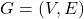. Show that for each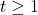, we have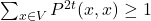. Moreover, if the graph is bipartite, then.

2.* Let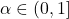. Consider a graphwhich consists of a complete graph onnodes, and a path of lengththat emanates from one of them (call it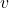) and ends in a node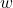. Give the best upper and lower bounds you can for

(a) The maximal hitting time for SRW in.

(b) The maximal hitting time for SRW started from.

(c) The cover time for SRW in.

(d) The mixing time for SRW in.

You can safely ignore constant factors, but pay attention to the dependence onand.

3. Read and understand the computation of the eigenvalues and eigenvectors for simple random walk on the-cycle.

## Reference

 Glasner, Shmuel.
Almost periodic sets and measures on the torus.
Israel J. Math. 32 (1979), no. 2-3, 161–-172.

 Berend, Daniel and Peres, Yuval.
Asymptotically dense dilations of sets on the circle.
J. London Math. Soc. (2) 47 (1993), no. 1, 1–-17.

 N. Alon and Y. Peres, "Uniform dilations'', Geometric and Functional Analysis, vol. 2, No. 1 (1992), 1–28.

 Nair, R. and Velani, S. L.
Glasner sets and polynomials in primes.
Proc. Amer. Math. Soc. 126 (1998), no. 10, 2835–-2840.